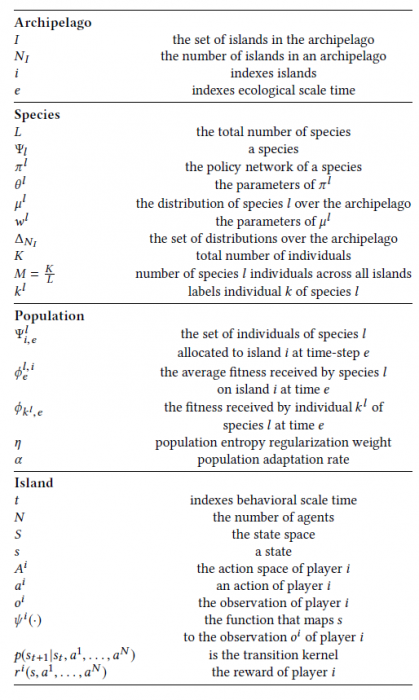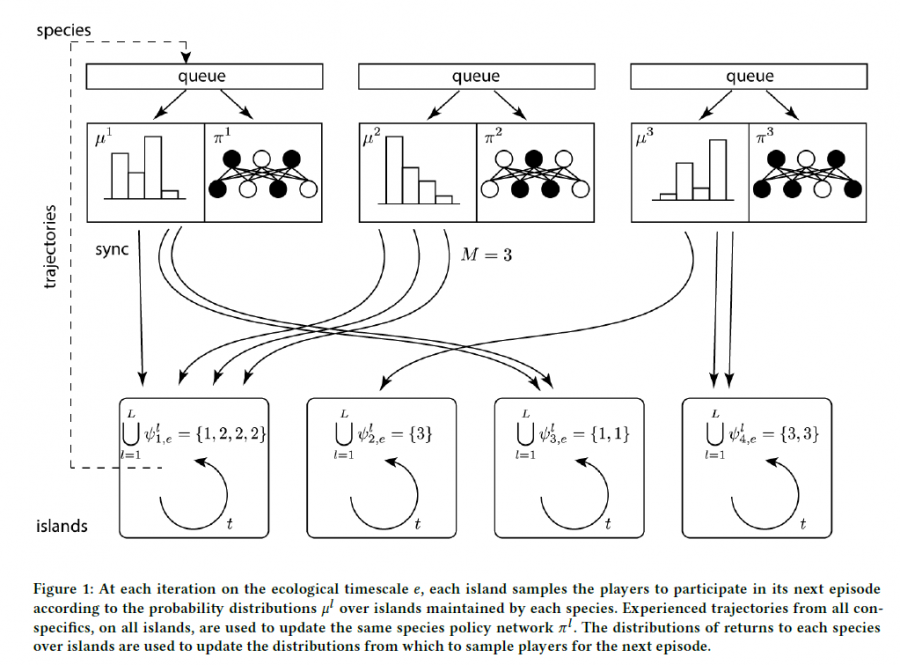# 多智能体强化学习算法

## Malthusian Reinforcement Learning

Posted by MY on August 10, 2020

## 一、问题

• 与基于内在动机的算法相比，马尔萨斯强化学习在避免陷入局部最优策略方面是否更好？
• 马尔萨斯强化学习中制定联合政策来实施异构共生行为是否比self-play的方法容易？

## 二、解法

• 马尔萨斯强化学习可以看作是“社区协同进化”的算法。它产生了一组社区，在本文中称为岛屿。每个岛屿都有一群执行策略的智能体。
• 每个个体都是一个物种的成员。同一物种的所有个体共享一个策略网络。
• 该算法在两个时间尺度上展开，分别对应于人口动态（生态）时间和策略执行（行为）时间。
• 人口动态与个体强化学习的回报相关。如果给定物种的个体在特定的岛屿上表现良好，那么将来该物种在该岛屿上的人口将会增加。
• 在每个episode中，给定物种的所有个体积累的经验用于v-trace算法训练公共神经网络。由特定物种的个体产生的经验仅用于更新自己的物种神经网络。在每个episode之后，每个物种在岛屿上的分布都会通过类似策略梯度的更新规则进行更新。
• 生物学上，现实的种群动态都包含指数增长的可能性。实际上，它们受到承载能力即环境特性的限制。为了确保可以使用有限的计算资源执行该操作，种群动态通过维护所有岛屿上每个物种的概率分布来进行。分配给任何给定岛屿的概率可能会根据在该岛获得的单个收益而增加或减少，但始终被约束为一个有效的概率分布。在任何给定的岛屿上，人口在每个episode都不同，但是在整个群岛中，个体的数量始终是恒定的。$\phi_{i, e}^{l}=\left(\sum_{k^{l} \in \Psi_{i, e}^{l}} \phi_{k^{l}, e}\right) /\left|\Psi_{i, e}^{l}\right| \text { and } 0 \text { if } \Psi_{i, e}^{l}=\emptyset$

$w_{e+1}^{l}=w_{e}^{l}+\alpha\left[\sum_{i \in\left\{1, \ldots, N_{I}\right\}} \nabla_{w^{l}} \mu^{l}(i)\left(\phi_{i, e}^{l}-\eta \log \mu^{l}(i)\right)\right]$

$\left[\sum_{i \in\left\{1, \ldots, N_{I}\right\}} \mu^{l}(i)\left(\phi_{i, e}^{l}-\eta \log \mu^{l}(i)\right)\right]$## 三、实验内容

• 互利双方的分工需要同一个网络表征，浪费网络的表征能力。
• 通过self-play的策略需要一种切换机制来确定在任何给定情况下要发出哪个子策略。切换策略的复杂性本身与环境中部分可观察性的程度有关。如果其他人的策略不容易被观察到，可能很难确定在任何给定时间（例如，互利双方关系）中执行互利关系各部分所需的个人的正确比例。
• 使用self-play学习一个不同的策略集合会更容易，每个策略都只实施其互利伙伴关系的一部分。但这必须要求预先知道每个策略副本的数量，从而增加了许多新的难以调整的超参数（每个物种一个）。

## 四、缺点

• 对于方法设置的描述不太清晰，人口学与生物学的例子讲得不够清楚
• 未使用最先进的遗传和进化算法。

## 五、优点

• 研究角度新颖，可以认为是开辟了一个新的研究方向
• 实验的理论分析非常充分，紧密结合了人口学和生物学的知识

##### Share